# 教程 | NumPy常用操作

NumPy 是 Python 语言的一个扩充程序库。支持高效的多数组与矩阵运算，此外也针对数组运算提供大量的数学函数库。NumPy 的科学计算十分高效，因此弥补了 Python 在运算效率上的不足。

import numpy as np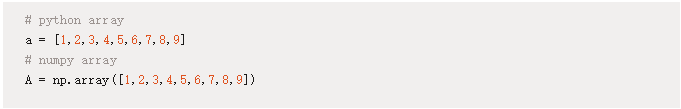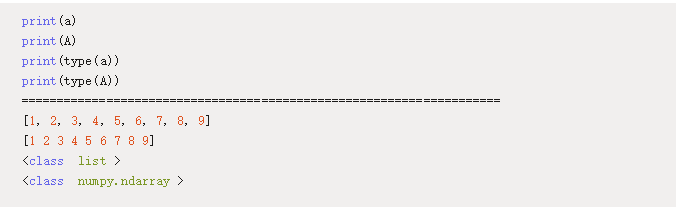np.arange()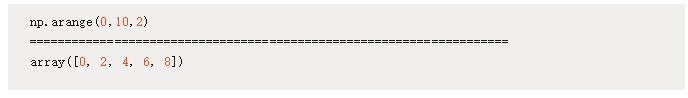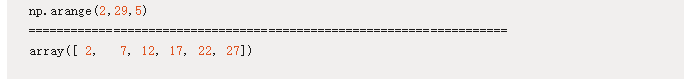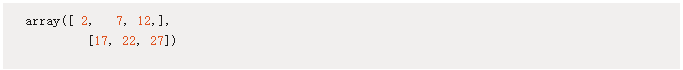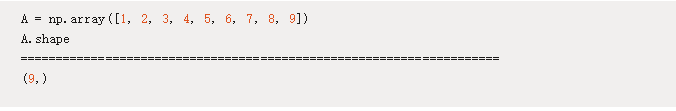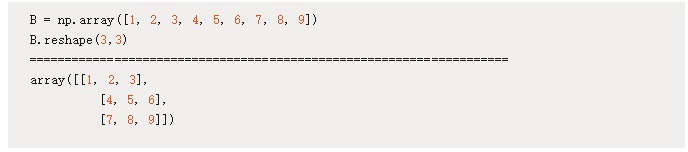np.zeros()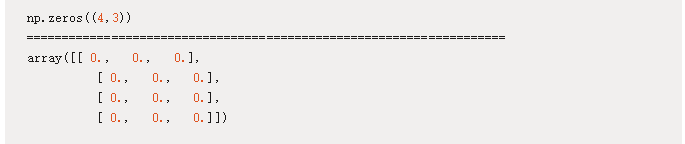np.zeros((n,m)) 将返回一个 n*m 阶矩阵，其中每个值都为零。

np.eye()

eye() 方法将生成一个单位矩阵：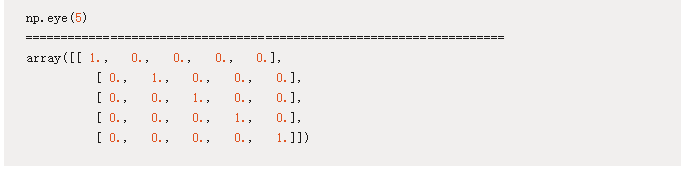np.eye(n) 将生成一个 n 阶单位方阵，即一个 n 阶矩阵，其主对角线元素都为 1，其它元素都为 0。

np.dot()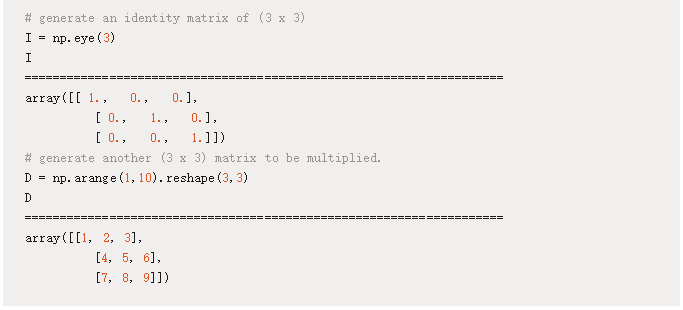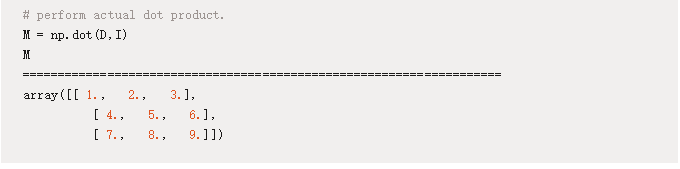np.sum()

np.sum() 会将整个矩阵的所有元素加和为一个标量值：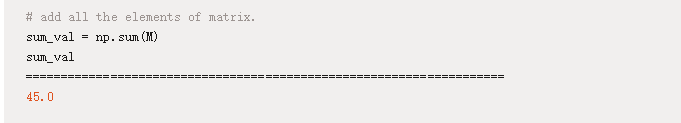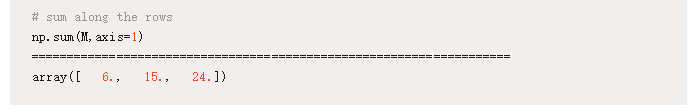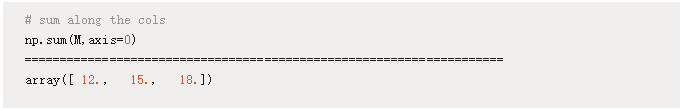np.random.rand()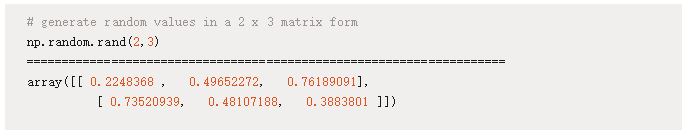np.append()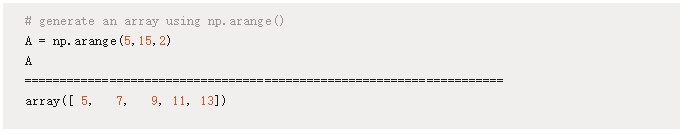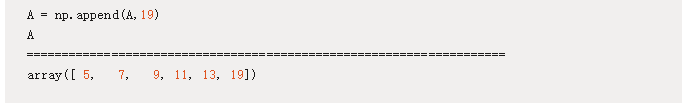np.append() 同样可以将一个具体的数组添加到已有的数组中：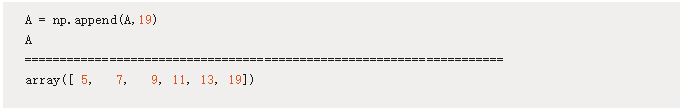np.diff()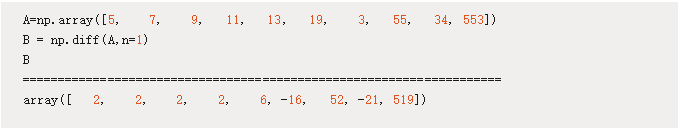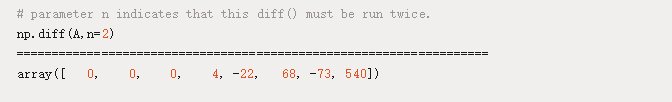np.vstack() 和 np.column_stack()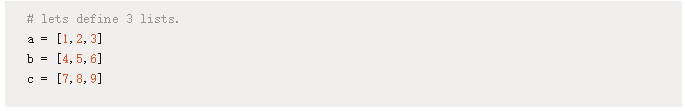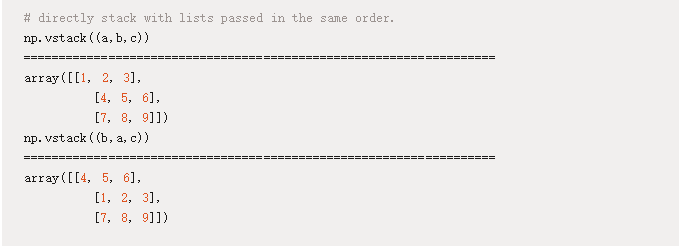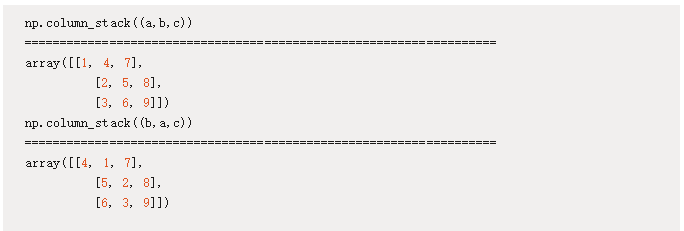np 数组索引

NumPy 数组的索引方式和 Python 列表的索引方式是一样的，从零索引数组的第一个元素开始我们可以通过序号索引数组的所有元素。例如 A[i] 索引数组 A 中的第 i+1 个元素。此外，我们还能索引一串元素：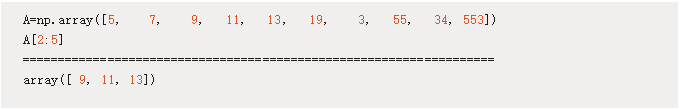A[lowerbound(inclusive): upperbound(exclusive)]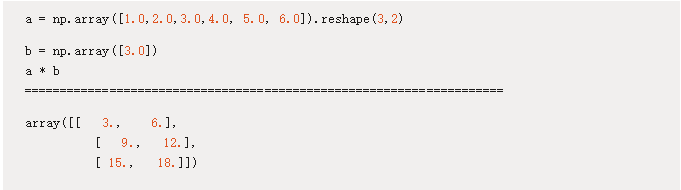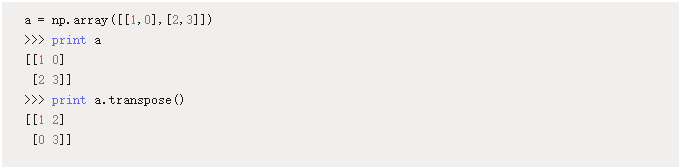nplg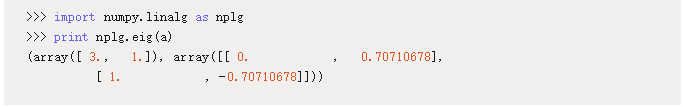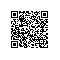使用钉钉扫一扫加入圈子
+ 订阅